## LaTeX | 为学术论文排版而生【公式篇】 转

#### 论数学公式

• Word中自带的插入公式功能。

• 借助Mathtype

☟，准备入坑！

#### LaTeX 数学公式语法

$a^2 + b^2 = c^2$• 字母是都知道，这不是问题。
• LaTeX编辑公式涉及到的符号特别多，先不说知道什么时候用哪个符号，你连把这些符号记住就不太可能了（累觉不爱，且行且珍惜：））

• $...$
• $$...$$
• \begin{math}...\end{math}

频率$f$等于速度$v$除以波长 $\lambda$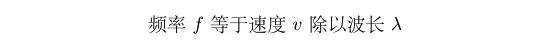• $$...$$
• $...$
• \begin{displaymath}...\end{displaymath}

$$f(x)= ax^2 + bx + c$$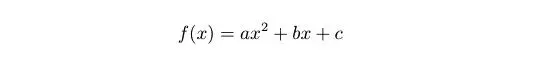\documentclass[UTF8]{ctexart}
\usepackage{amsmath}
\begin{document}

\begin{equation}
f(x)= ax^2 + bx + c
\end{equation}

\end{document}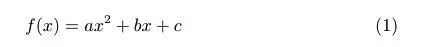\documentclass[UTF8]{ctexart}
\usepackage{amsmath}
\numberwithin{equation}{section}
\begin{document}

\section{Math}
\begin{equation}
f(x)= ax^2 + bx + c
\end{equation}

\end{document}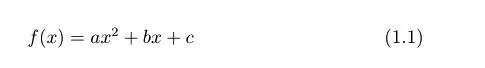#### 你可能不知道的 MathType 功能

• 首先在MatyType中编辑好自己需要的公式，比如二次函数根的表达式：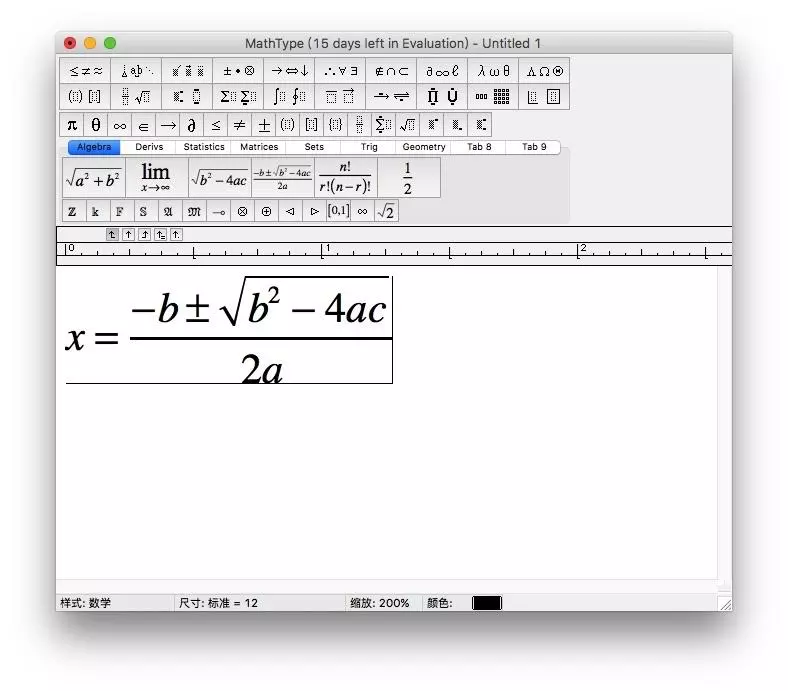编辑公式

• 在菜单栏选择剪切并拷贝预置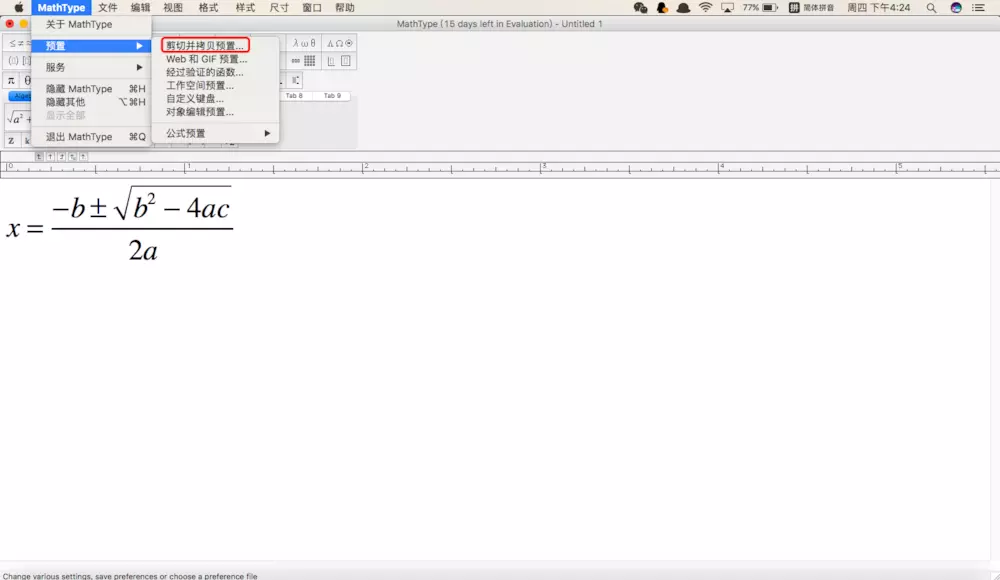MathType设置

• 选择MathMl 或 TeX，点击确定，完成设置。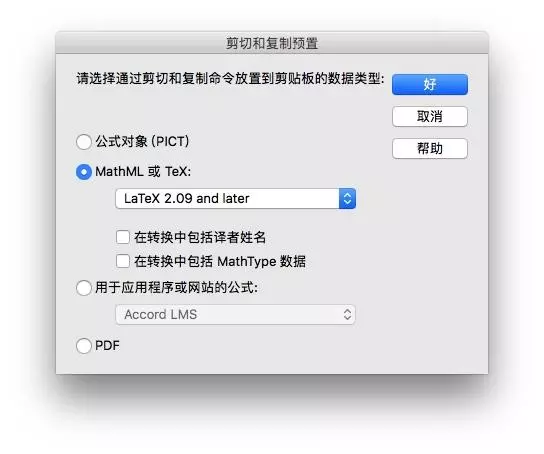MathType设置

• 然后全选编辑器好的公式，Ctrl+C复制。

• LaTeX编辑器（比如TeXstudio）粘贴，得到：

$x = \frac{{ - b \pm \sqrt {{b^2} - 4ac} }}{{2a}}$



• 编译代码得到：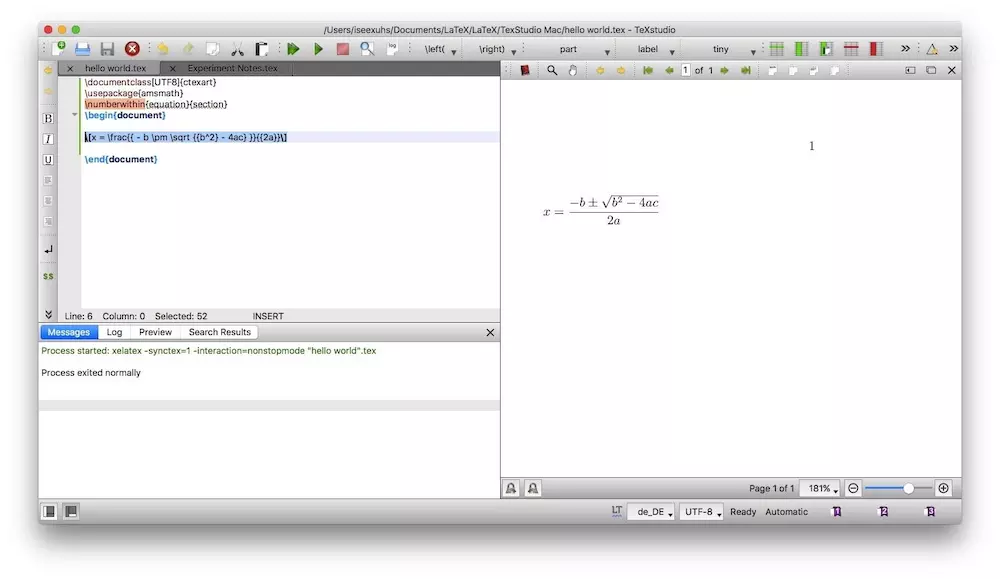编译输出

• 到此完成。

Paper_weekly
2018/09/08
0
0
LaTeX | 为学术论文排版而生【浮动体篇】

2018/12/22
137
0
LaTeX | 为学术论文排版而生【文本篇】

2018/12/22
92
0
LaTeX | 为学术论文排版而生【入门篇】

2018/12/22
65
0

2018/11/24
0
0

zzxworld
44分钟前
5
0
sonar报错volatile

49分钟前
4
0
《Java实践指南》--读后

RippleChan
50分钟前
4
0

52分钟前
6
0

3
0